Study Guide

# Special Relativity Questions

## Special Relativity Questions

1. Galilean transformations assume time is absolute as opposed to relative. As we've discovered, this doesn't work for speeds approaching the speed of light. How would Galilean transformations change taking special relativity into account? Proceed by remembering two things: first, the Lorentz-Einstein transformations should reduce to Galilean transformations at non-relativistic speeds (v << c) and second, the speed of light should remain constant in any frame of reference.

This question is basically asking us to derive the Lorentz-Einstein transformations, which we brought up in Section 2.4. Those are:

x' = x − vt
y' = y
z' = z
t' = t

Let's try a generic form of x' = γ(x − vt) if S' is moving in the positive x direction relative to S, and x = γ(x' + vt) if it's the other way around. Let's pretend we don't know what γ is but that it's dependent on v.

We need to be sure the speed of light is constant in both S and S' frames. To do this, we use our sphere of light again (the one we used to derive our spacetime interval). Imagine a frame of reference S at rest with coordinates (x, t). Another frame of reference S' with coordinates (x', t') comes flying by with velocity relative to S. Both frames intersect at their origins. At that precise moment, a light sparks at t = 0 and spreads out with a radius equal to ct. Because the speed of light is always constant, x = ct and x' = ct'. If we plug that into our experimental x and x' equations, we get that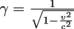,as we should.

We now substitute γ into x = γ(x' + vt) and x' = γ(x − vt) and to solve for t, and we get that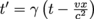and .

The equations all reduce to Galilean transformations at non-relativistic speeds of v << c.

2) From the above relativistic transformations, determine how to obtain relativistic velocity addition equations using the basic definition of velocity,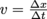.

Velocity is always given by the change of position over the change of time. What we can do is designate two different positions, x2 and x1, and write them out with our relativistic transformations.

Using x = γ(x' + vt) , we get that Δx = x2 x1 = γ[(x2' x1') + v(t2' t1')] = γx' + vΔt').
Using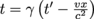, we find that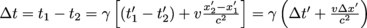.

With these two equations for Δx and Δt, we can finally form our velocity ux: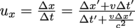.This simplifies to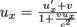,and by similar process we also find that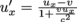3. An alien signal moves past Earth at a speed of c. Hoping to decipher the message, we jump into a ship that travels at 0.8c. Using relativistic velocity addition, show that the speed of light c of the alien signal doesn't change according to our spaceship.

Our relativistic velocity addition equations are given byand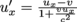The question asks us to compare ux and ux'. If we pick Earth's frame as S, then we have that ux is c and v is v = 0.8c. Back to our equation, we get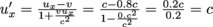.

4. As we've read, two physicists conducted an experiment in 1941 that showed the effects of time dilation on relativistic particles, particularly μ-mesons interacting with Earth's atmosphere. The experiment took place on Mount Washington in New Hampshire, which has an altitude of 2000 m. Knowing that muons hit the top of the mountain at an average rate of 560 muons per hour and a velocity of v = 0.9994c, use relativity to show the exact impact of time dilation on muon survival rate.

Newtonian mechanics predict that muons will travel a distance of d = 2000 m at v = 0.9994c in a period of time = 6.7 μs. In this interval, only about 25 muons are expected to survive the trip using their radioactive decay rate.

Taking relativity into effect, we can calculate proper time for the muons' trip. Plugging in v = 0.9994c into our relativistic factor equation, and using Δt = γΔto, we can solve for Δto and get that Δto = 0.73 μs. In that amount of time, radioactive decay laws predict that 414 muons will reach the bottom of the mountain, which was exactly the measured number.

5. A twin named Alice leaves Earth and travels to a distant star at a speed of 0.8c for 20 years. Discuss the effects of time dilation and length contraction using special relativity, taking into account her twin named Bob who stays on Earth.

A velocity of v = 0.8c will give a gamma factor of γ = 1.67. Proper time here Alice's time, or Δto = 20 years. Bob's time can then be found with Δt = γΔto = 1.67 × 20 = 33.4 years. When Alice comes back to Earth, Bob will be 13.4 years older than her.
Now let's analyze this with length contraction, measuring the distance Alice travels in the length of lightyears, or how far light travels in a year's time. According to Alice, she travels a distance of Δl= vΔto = (0.8c)(20 years) = 16 light years. According to Bob, she travels a distance Δlo = vΔt = (0.8c)(33.4 years) = 26.72 light years. According to Albert Einstein, the proper length or distance should be given by Δlo= γΔlo.
Alice's traveled distance, Δl, would be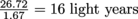, which works out perfectly with our predictions. Bravo.

6. The above scenario designates Alice as the one traveling in a proper frame of reference, and the one whose life span gets expanded. Yet we could also very well say that she's at rest while her twin Bob on Earth travels for 20 years in the other direction at a speed of -0.8c. On top of this, Einstein's postulates insist we can't pick a frame of reference over another, so both frames of reference should be analyzed equally. So who turns out to be the youngest twin here? Alice or Bob? Discuss this twin paradox keeping the definition of special relativity in mind.

By definition, special relativity only applies to inertial frames of references– they are either at rest or moving with constant velocity. However, Alice has to accelerate when she decides to around and return to Earth. This means she feels an inertial force and Bob doesn't. Although we can't tell which twin is really traveling, the scenario is not longer symmetrical, and Alice really is the one who experiences time dilation. Lucky her.

7. Expand on the twin paradox by considering the possibility that Alice never turns around.

If Alice never turns around, then she doesn't have to accelerate and never feels inertial forces when returning back home. Then can't we argue that the situations are back to being symmetrical, and Bob could very well claim he's the one who's been traveling for 20 years? In theory, yes. We could set up synchronized clocks on Alice's ship and in Bob's office on Earth and compare readings when Alice's ship passes Bob's office, or vice-versa.

The problem is that synchronized clocks according to Alice's ship wouldn't be synchronized according to Bob. We've seen this before as events being simultaneous in one frame, and not simultaneous in another.

It works out either way. For twins to compare clocks, or see who's aged more, they have to rendezvous at some point. This means one of them will have to experience inertial forces to reach the other, breaking the symmetry once again.

8. Explore the relativistic expression for total energy E by checking what happens when p is extremely large.

Energy is given by E2 = p2c2</em> + (moc2</em>)<em>2</em> = γ2mo2c2. When p >> c, then p2c2</em> >> (moc2</em>)<em>2</em>. The expression reduces to what we could expect for a photon, E = pc.
9. Explore the relativistic expression for total energy E by checking what happens when p is extremely small

What happens when p << c? We can use the definition of the relativistic factor and solve in terms of p and u. Knowing that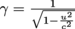and that the momentum squared is given by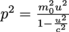,we can get an expression for γ in terms of p, such that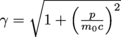E2 = γ2mo2c2 results in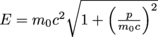.

ince u << c, our fraction of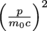is much less than 1. This means we can use the binomial theorem12 and expand our square root as (a+b)<em>n</em>~ an</em>+ na(<em>n</em>-1)b. Assigning a = 1,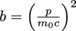, and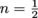,we get a final expression for energy consisting of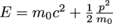,which is equivalent to the rest energy plus kinetic energy.

10. Einstein wrote a paper in 190513, which analyzed how the position of two clocks on the Earth, one at the North pole and one at the equator, would affect their reading. Explain how he reached this conclusion.

Let's assume the pole is at rest and measures the Earth's motion with time Δt. We can also approximate the motion of the equator for a short period of time Δto as motion in a straight line with constant speed. The idea behind this is to find this speed, and then use it to calculate a difference in times, both at the pole and at the equator.

So– what is this rotational speed? Earth rotates on its axis and makes a full rotation in 24 hours. A full rotation is 360 degrees, or 2π. The Earth's angular velocity w is then given by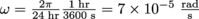.

Since the radius of the Earth is given by R = 6378 km14, we can get a value for linear velocity v, as v = Rw = 6378 km × (7 × 10-5) ~ 0.46 km per sec. This gives a beta of β = 2.35 × 10-12. Solving for Δto and Δt won't differ much since β is so low. Instead, let's try to get an expression for Δt − Δto.
Since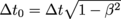,we can write that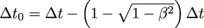, such that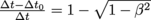.Using the binomial theorem once again, we get simplify the equation to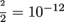.Therefore, the times at the equator and the pole will differ by a small amount of 10-12 due to time dilation.

11. Investigate how the quantity of momentum needed to accelerate a particle to relativistic speeds increases drastically as the particle moves faster and faster.

We know the momentum p of a particle is given by p = γmu. Writing a table for other speeds ranging from u = 0 to u = 0.99cwill let us see how the momentum is directly affected.
p(0c) = 0
p(0.001c) = 0.001mc
p(0.1c) = 1.01mc
p(0.2c) = 1.02mc
p(0.3c) = 1.05mc
p(0.4c) = 1.09mc
p
(0.5c) = 1.15mc
p
(0.6c) = 1.25mc
p
(0.7c) = 1.4mc
p
(0.8c) = 1.67mc
p
(0.9c) = 2.29mc
p
(0.95c) = 3.20mc
p
(0.99c) = 7.09mc

From these values, you can judge for yourself how momentum increases so differently. For a speed of 0.3c and 0.4c, we get momentum values of 1.05mc and 1.09mc. A 0.1c difference at low speeds only gives us a momentum difference of 0.04mc. But for speeds much higher, say 0.8c and 0.9c, we get momentum values of 1.67mc and 2.29mc. That's a momentum difference of 0.62mc, which is much larger than the first tenth of c jump.